# Mean Motion Resonances HamiltonJacobi equation and Hamiltonian formulation

• Slides: 52
Download presentationMean Motion Resonances Hamilton-Jacobi equation and Hamiltonian formulation for 2 -body problem in 2 dimensions Cannonical transformation to heliocentric coordinates for N-body problem Symplectic integrators Canonical transformation Orbital trajectories of Jupiter's four largest moons over a period of 10 Earth with resonant angle days, illustrating the 4: 2: 1 resonance Mean motion resonances among the orbits of the inner three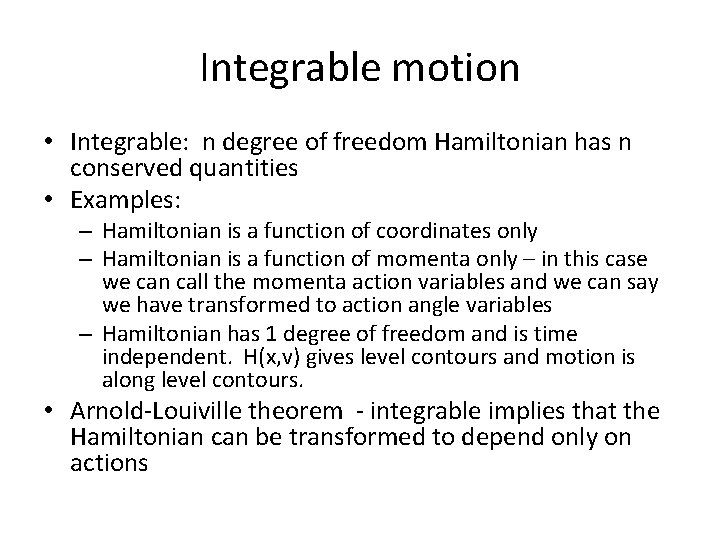Integrable motion • Integrable: n degree of freedom Hamiltonian has n conserved quantities • Examples: – Hamiltonian is a function of coordinates only – Hamiltonian is a function of momenta only – in this case we can call the momenta action variables and we can say we have transformed to action angle variables – Hamiltonian has 1 degree of freedom and is time independent. H(x, v) gives level contours and motion is along level contours. • Arnold-Louiville theorem - integrable implies that the Hamiltonian can be transformed to depend only on actionsHamilton Jacobi equation • If the coordinate q does not appear in the Hamiltonian the corresponding momentum p is constant • Try to find a Hamiltonian that vanishes altogether, then everything is conserved • Generating function S 2(q 1, q 2, …; P 1, P 2, …, t) function of old coordinates and new momenta which we would like conserved • New Hamiltonian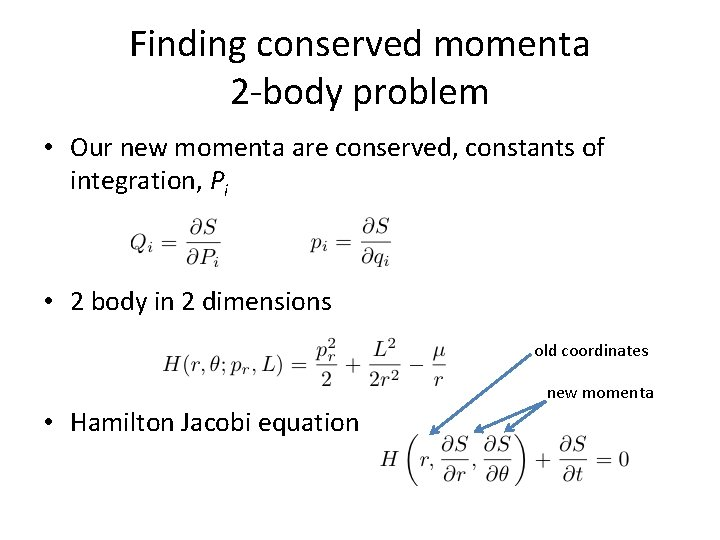Finding conserved momenta 2 -body problem • Our new momenta are conserved, constants of integration, Pi • 2 body in 2 dimensions old coordinates new momenta • Hamilton Jacobi equation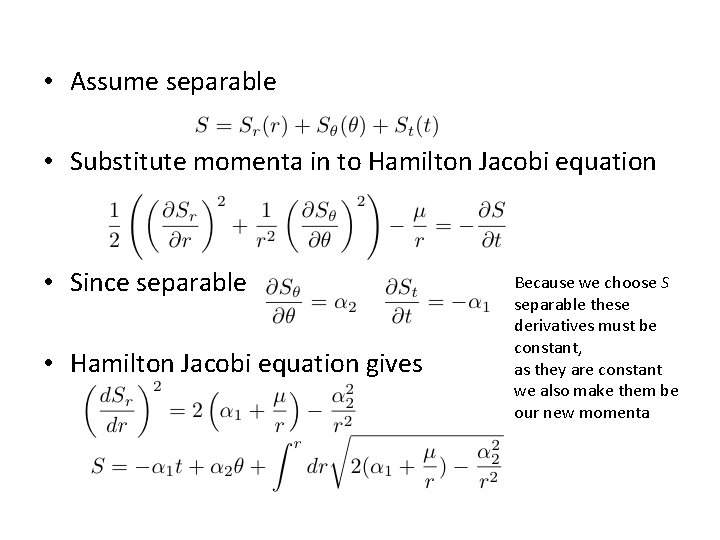• Assume separable • Substitute momenta in to Hamilton Jacobi equation • Since separable • Hamilton Jacobi equation gives Because we choose S separable these derivatives must be constant, as they are constant we also make them be our new momenta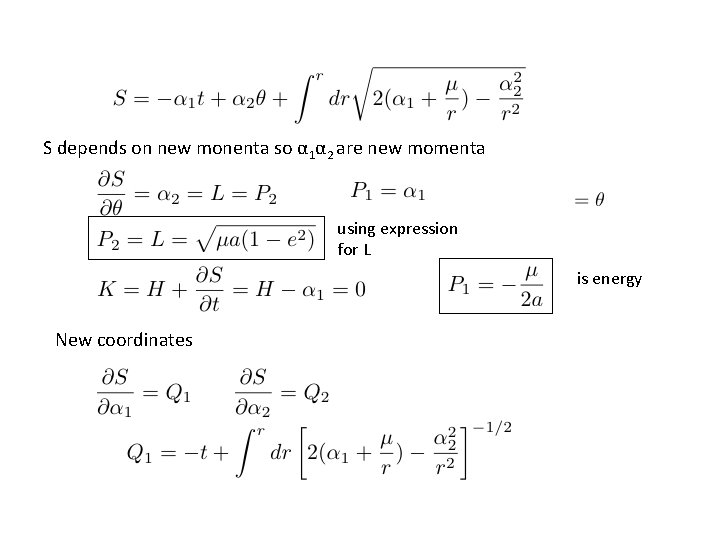S depends on new monenta so α 1α 2 are new momenta using expression for L is energy New coordinates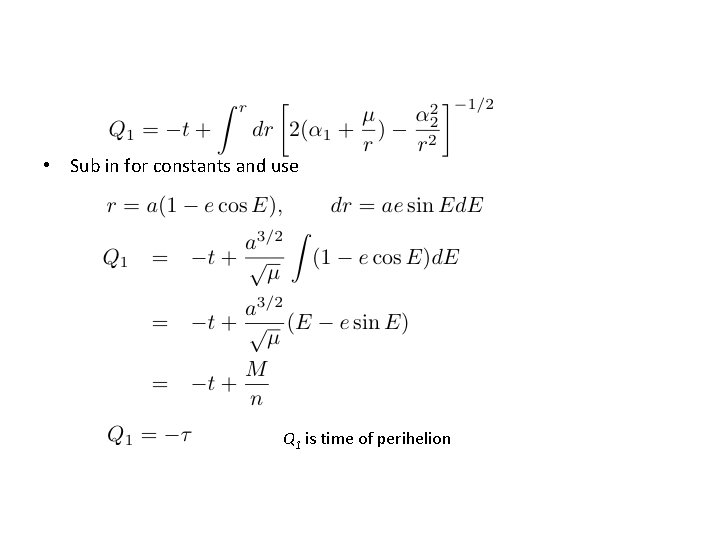• Sub in for constants and use Q 1 is time of perihelion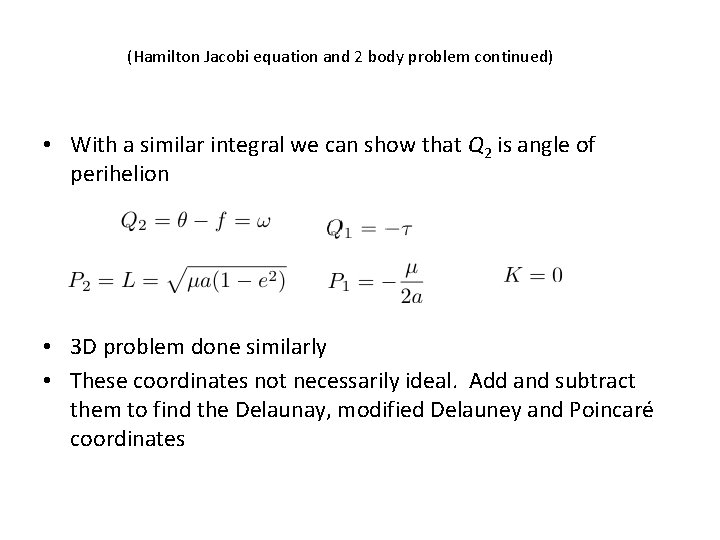(Hamilton Jacobi equation and 2 body problem continued) • With a similar integral we can show that Q 2 is angle of perihelion • 3 D problem done similarly • These coordinates not necessarily ideal. Add and subtract them to find the Delaunay, modified Delauney and Poincaré coordinatesCannonical transformations Different approaches 1. Choose desirable generating functions 2. Solve integrals resulting from Hamilton. Jacobi equation 3. Choose new coordinates and momenta and show they satisfy Poisson brackets 4. Use expansions (e. g. , Birkhoff normal form) (can lead to problems with small divisors)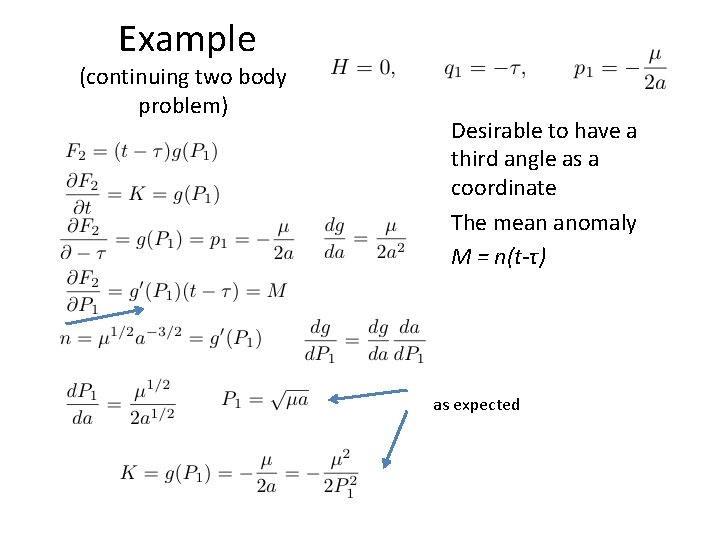Example (continuing two body problem) Desirable to have a third angle as a coordinate The mean anomaly M = n(t-τ) as expected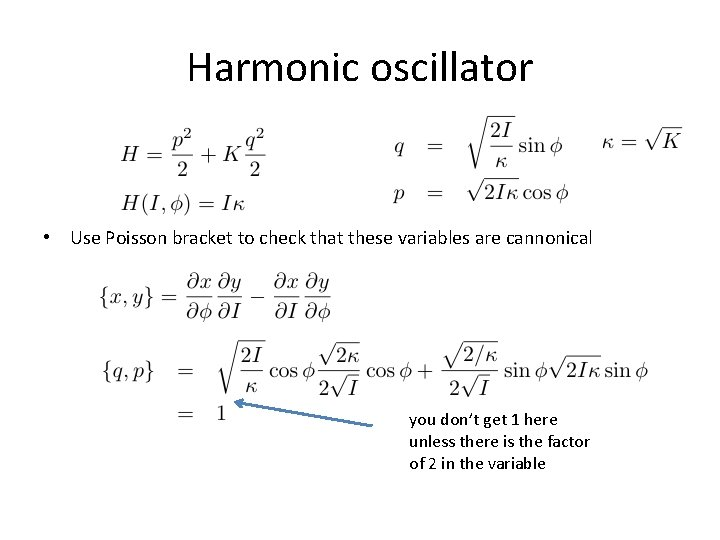Harmonic oscillator • Use Poisson bracket to check that these variables are cannonical you don’t get 1 here unless there is the factor of 2 in the variableHarmonic oscillator Using a generating function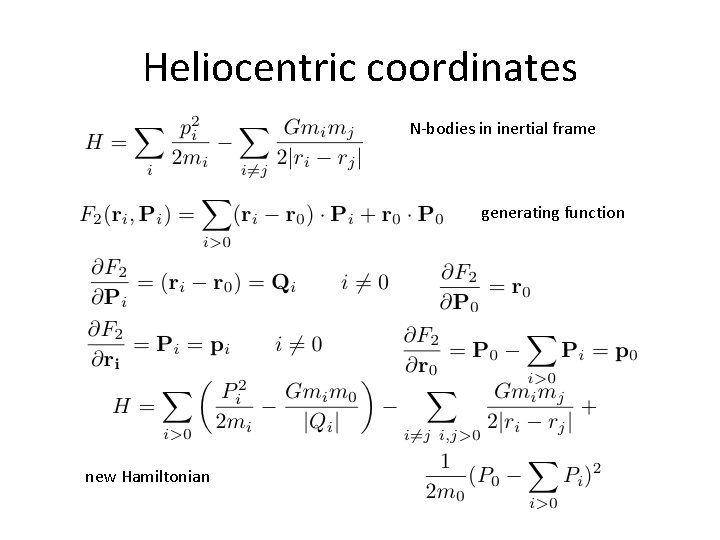Heliocentric coordinates N-bodies in inertial frame generating function new HamiltonianDemocratic heliocentric coordinate system barycenter so can be set to zero This is equivalent to using momenta that are in center of mass coordinate system • If P 0=0 Hamiltonian becomes Keplerian term Interaction term Drift termDemocratic heliocentric coordinates • Hamiltonian is nicely separable in – heliocentric coordinates and – barycentric momentaJacobi Coordinates To add a body: work with respect to center of mass of all previous bodies. Coordinate system requires a tree to define.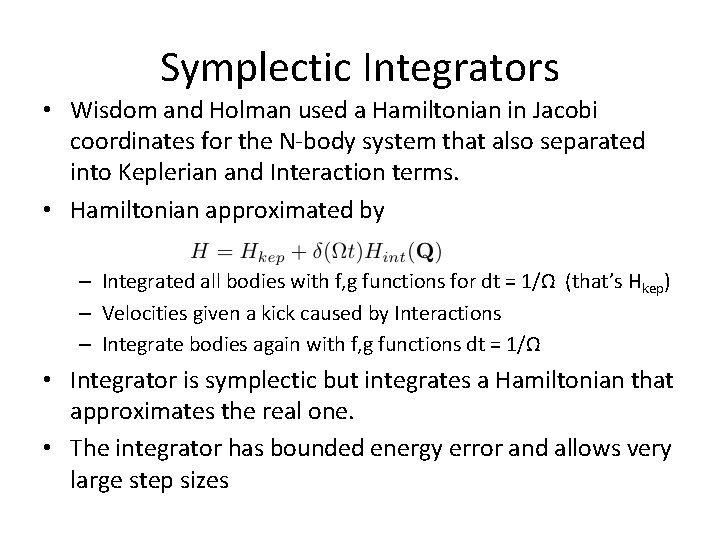Symplectic Integrators • Wisdom and Holman used a Hamiltonian in Jacobi coordinates for the N-body system that also separated into Keplerian and Interaction terms. • Hamiltonian approximated by – Integrated all bodies with f, g functions for dt = 1/Ω (that’s Hkep) – Velocities given a kick caused by Interactions – Integrate bodies again with f, g functions dt = 1/Ω • Integrator is symplectic but integrates a Hamiltonian that approximates the real one. • The integrator has bounded energy error and allows very large step sizesSecond order Symplectic integrator Poisson bracket with H gives evolution Find coefficients so this is true to whatever order you desire (see Yoshida review)Second order Symplectic integrator Expand to second order ~ =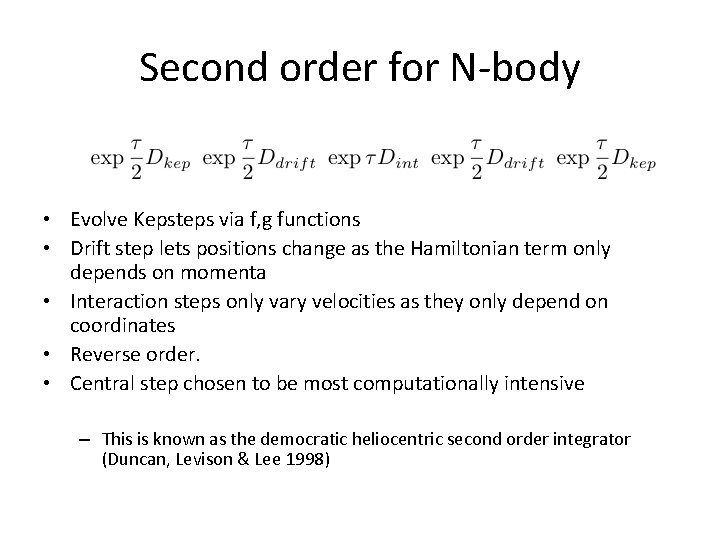Second order for N-body • Evolve Kepsteps via f, g functions • Drift step lets positions change as the Hamiltonian term only depends on momenta • Interaction steps only vary velocities as they only depend on coordinates • Reverse order. • Central step chosen to be most computationally intensive – This is known as the democratic heliocentric second order integrator (Duncan, Levison & Lee 1998)Symplectic integrators- Harmonic oscillator • The exact solution is • To first order in τ • However and energy increases with time • A symplectic scheme can be constructed with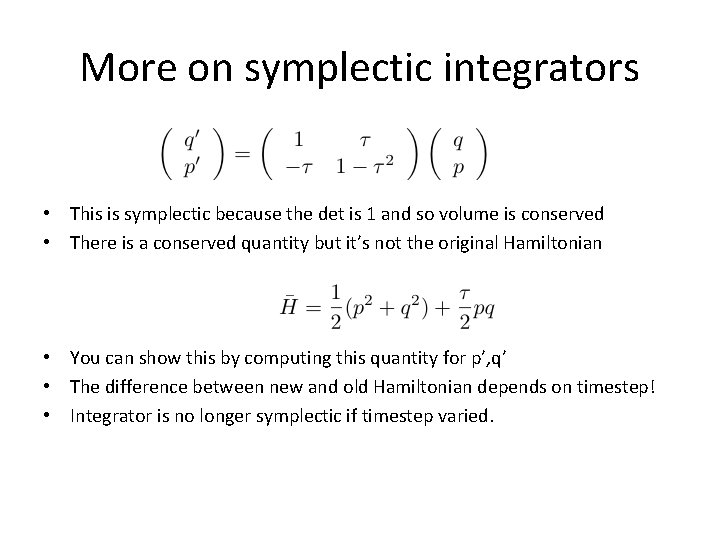More on symplectic integrators • This is symplectic because the det is 1 and so volume is conserved • There is a conserved quantity but it’s not the original Hamiltonian • You can show this by computing this quantity for p’, q’ • The difference between new and old Hamiltonian depends on timestep! • Integrator is no longer symplectic if timestep varied.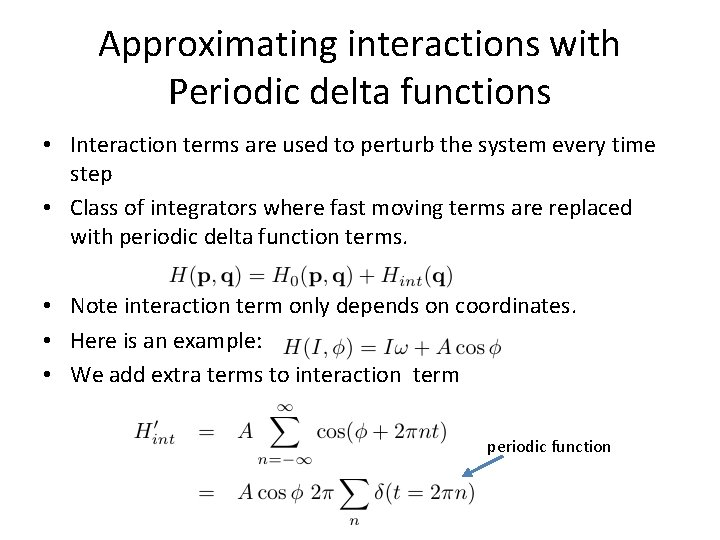Approximating interactions with Periodic delta functions • Interaction terms are used to perturb the system every time step • Class of integrators where fast moving terms are replaced with periodic delta function terms. • Note interaction term only depends on coordinates. • Here is an example: • We add extra terms to interaction term periodic functionIntegrating the approximate Hamiltonian • For t≠ 2πn • For t=0, 2π, 4π… • Integrate over the delta function • Procedure: integrate unperturbed Hamiltonian between delta function spikes. At each t=0, 2π, 4π… update momenta. These are the velocity kicks.Justifications • Often the Hamiltonian has many cosine terms. As long as frequencies are not commensurate, a perturbative transformation can remove non-resonant terms. Dynamics is only weakly sinusoidally varied by these terms. Cosine terms that are not commensurate are ignored. • We can add in cosine terms without significantly changing the dynamics. • The approximate Hamiltonian is conserved exactly. • Sizes of errors can be quantified. Errors are bounded as there is a conserved quantity. • You can’t change the step size as this would change the approximate Hamiltonian integrated. If you change the step size, the bounded property of errors is lost. • Wisdom, Holman, and collaborators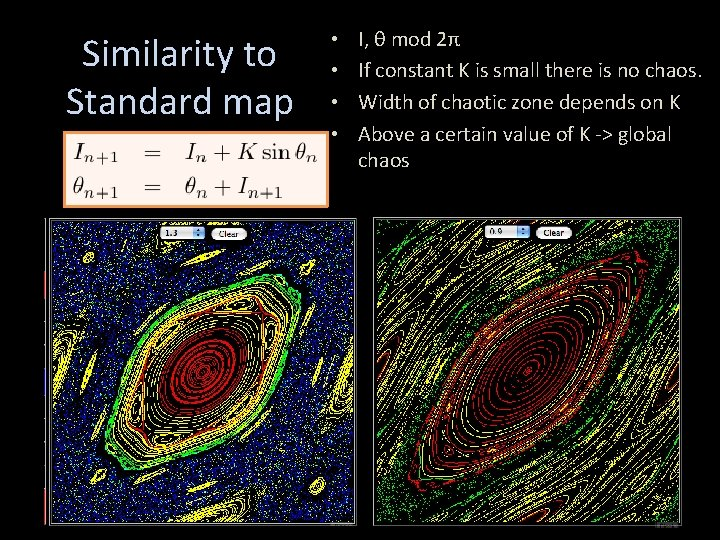Similarity to Standard map • • I, θ mod 2π If constant K is small there is no chaos. Width of chaotic zone depends on K Above a certain value of K -> global chaos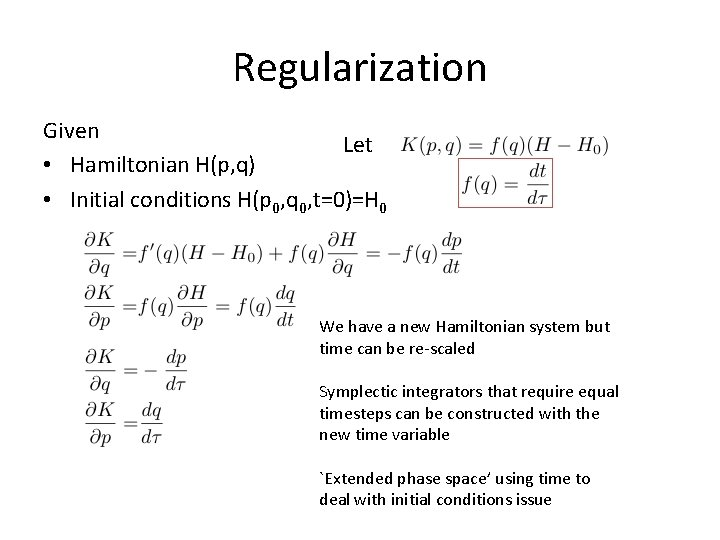Regularization Given Let • Hamiltonian H(p, q) • Initial conditions H(p 0, q 0, t=0)=H 0 We have a new Hamiltonian system but time can be re-scaled Symplectic integrators that require equal timesteps can be constructed with the new time variable `Extended phase space’ using time to deal with initial conditions issueGeneral view of resonance vector of integers k such that Contrast with Periodic orbits a period T such that for every frequency ωi is close to an integer Zi T is a multiple of the period of oscillation for every angleSmall divisor problem frequencies expand perturbation in Fourier series We would like to find new variables similar to the old variables try this and try to find nice values for ck Canonical transformation Insert this back into Hamiltonian Choose These can be small!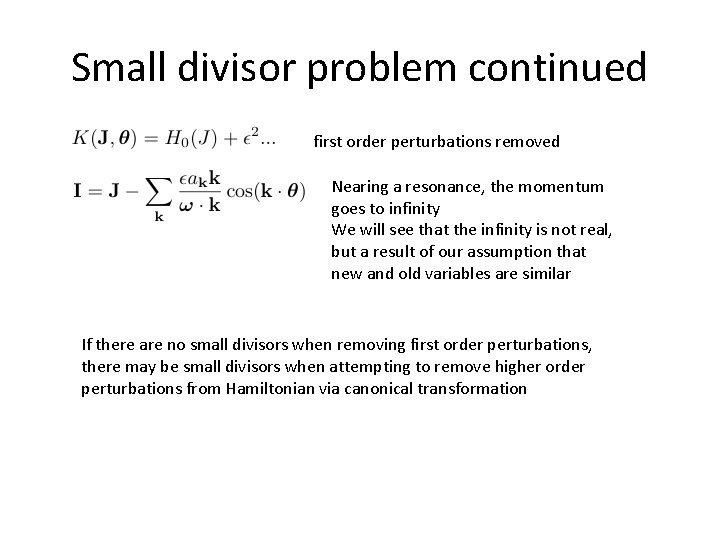Small divisor problem continued first order perturbations removed Nearing a resonance, the momentum goes to infinity We will see that the infinity is not real, but a result of our assumption that new and old variables are similar If there are no small divisors when removing first order perturbations, there may be small divisors when attempting to remove higher order perturbations from Hamiltonian via canonical transformationUsing the resonant angle generating function resonant angle is a new coordinate n-1 conserved quantities Ji because Hamiltonian lacks associated angles Can expand H 0 in orders of J 0Resonant angle Simple 2 D Hamiltonian. There is no infinity in the problem. Dynamics is similar to that of the pendulum. We did not assume that all new angles were similar to old angles in the transformation. The infinite response previously seen near resonance was caused by the choice of coordinate system Above we considered only a single cosine term When there is more than one cosine term, then dynamics can be more complicated Adopt a condition that the system is always sufficiently far from resonance, allowing perturbation theory to be done at all orders (Kolmogorov approach) Consider proximity of resonances (Chirikov approach)Expanding about a mean motion resonance (Celestial mechanics) • j: j-k resonance exterior to a planet unperturbed Hamiltonian units μ=1 • generating function mean longitude of planet • new momenta ψ is resonant angleExpand around resonance with coefficients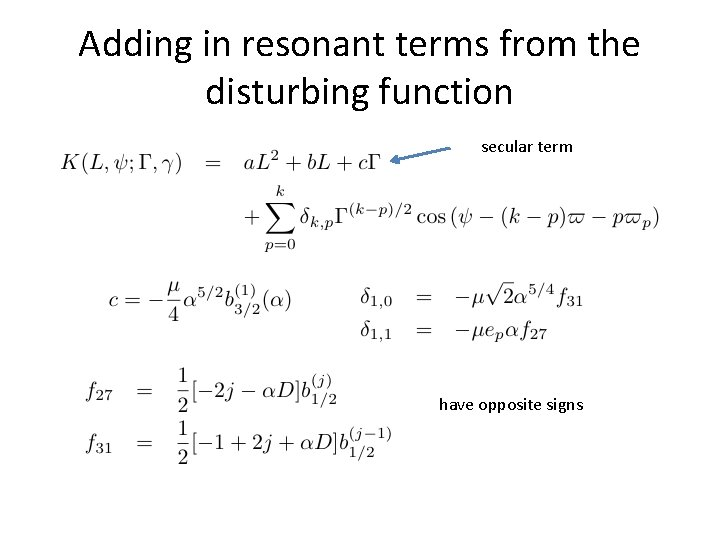Adding in resonant terms from the disturbing function secular term have opposite signsFirst order resonances sets distance to resonance corotation term proportional to planet eccentricity usually dropped but can be a source of resonance overlap + chaos (Holman, Murray papers in 96)One last C-transformation and going down a dimension New Hamiltonian has no second angle so J 2 is conserved We can ignore it in dynamics It may be useful to remember J 2 later to relate changes in eccentricity to changes in semi-major axis in the resonance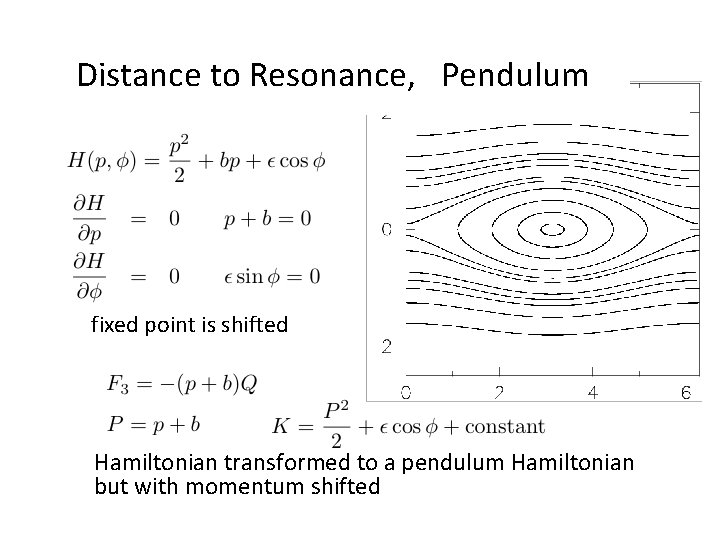Distance to Resonance, Pendulum fixed point is shifted Hamiltonian transformed to a pendulum Hamiltonian but with momentum shifted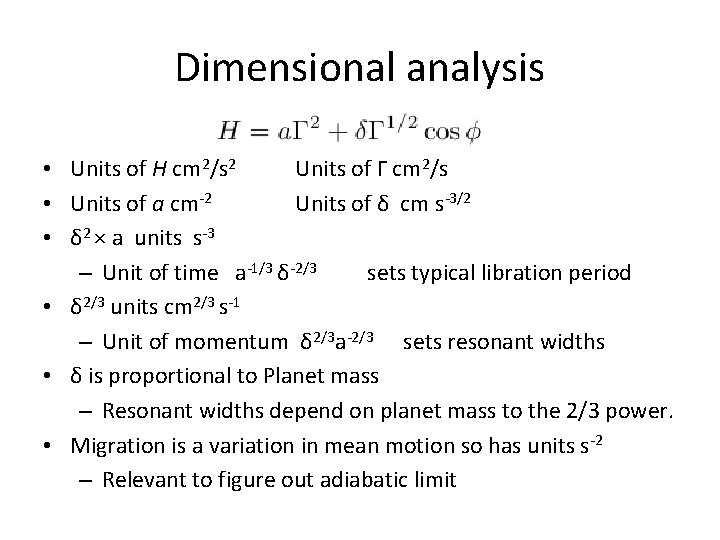Dimensional analysis • Units of H cm 2/s 2 Units of Γ cm 2/s • Units of a cm-2 Units of δ cm s-3/2 • δ 2 × a units s-3 – Unit of time a-1/3 δ-2/3 sets typical libration period • δ 2/3 units cm 2/3 s-1 – Unit of momentum δ 2/3 a-2/3 sets resonant widths • δ is proportional to Planet mass – Resonant widths depend on planet mass to the 2/3 power. • Migration is a variation in mean motion so has units s-2 – Relevant to figure out adiabatic limitAs distance to resonance varied coordinate system • Only one side has a separatrix • On one side it looks like a harmonic oscillator • 3 fixed points but only 2 stable • Without circulating about origin means in resonance • Can think of drifting problems as having time dependent b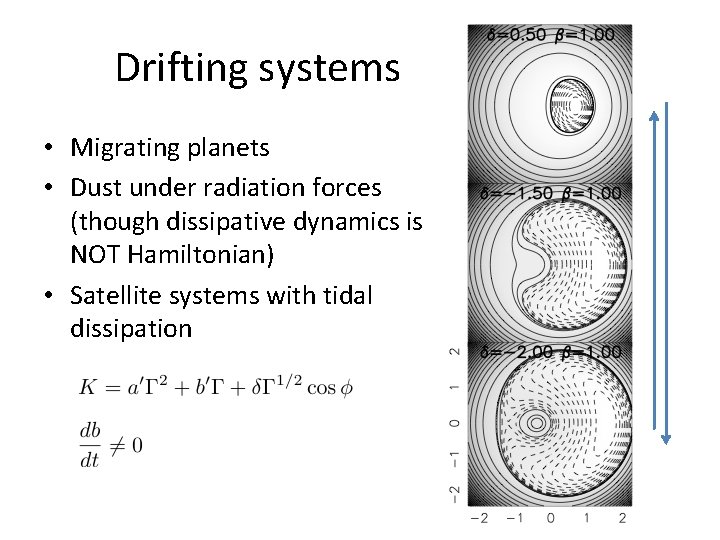Drifting systems • Migrating planets • Dust under radiation forces (though dissipative dynamics is NOT Hamiltonian) • Satellite systems with tidal dissipation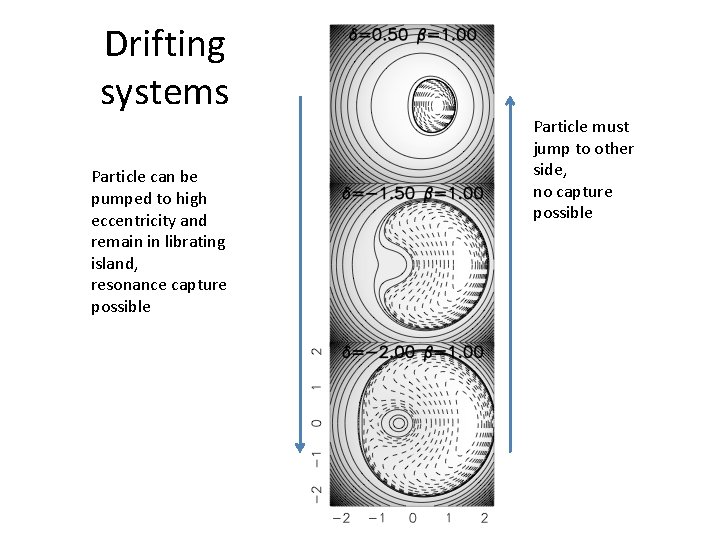Drifting systems Particle can be pumped to high eccentricity and remain in librating island, resonance capture possible Particle must jump to other side, no capture possible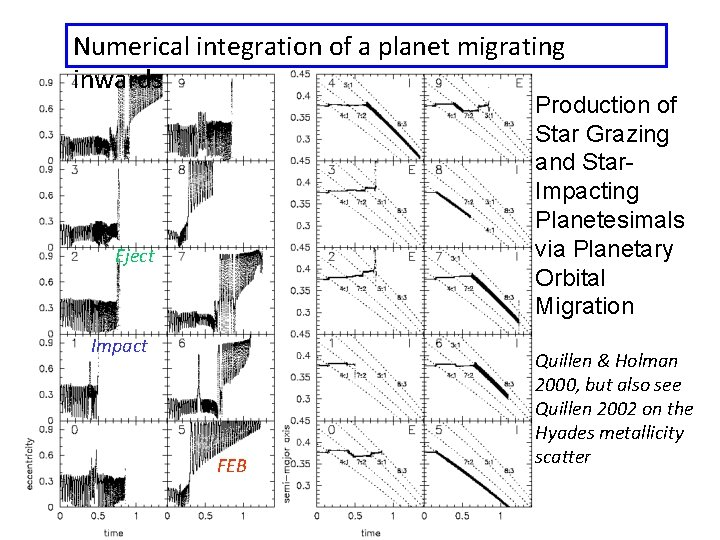Numerical integration of a planet migrating inwards Production of Star Grazing and Star. Impacting Planetesimals via Planetary Orbital Migration Eject Impact FEB Quillen & Holman 2000, but also see Quillen 2002 on the Hyades metallicity scatterIntegration with toy model resonant angle fixed -Capture EscapeFailure to capture • Volume in resonance shrinking rather than growing (particle separating from planet) • Non-adiabatic limit. Rapid drifting • Initial particle eccentricity is high. • Prevented by strong subresonances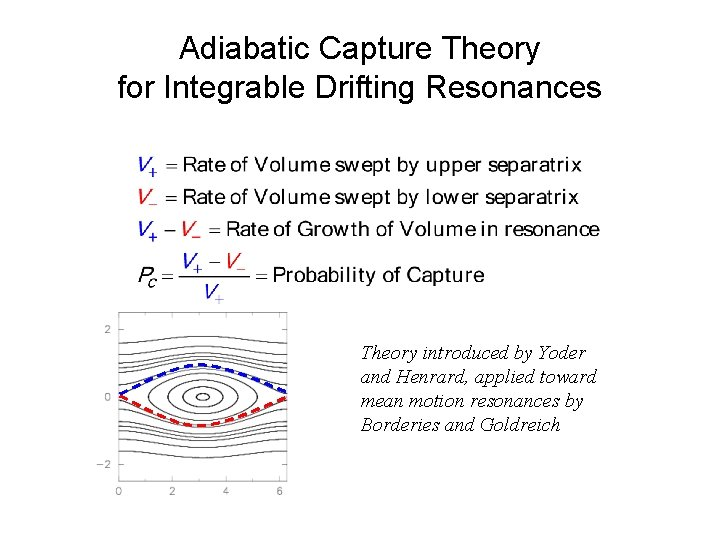Adiabatic Capture Theory for Integrable Drifting Resonances Theory introduced by Yoder and Henrard, applied toward mean motion resonances by Borderies and Goldreich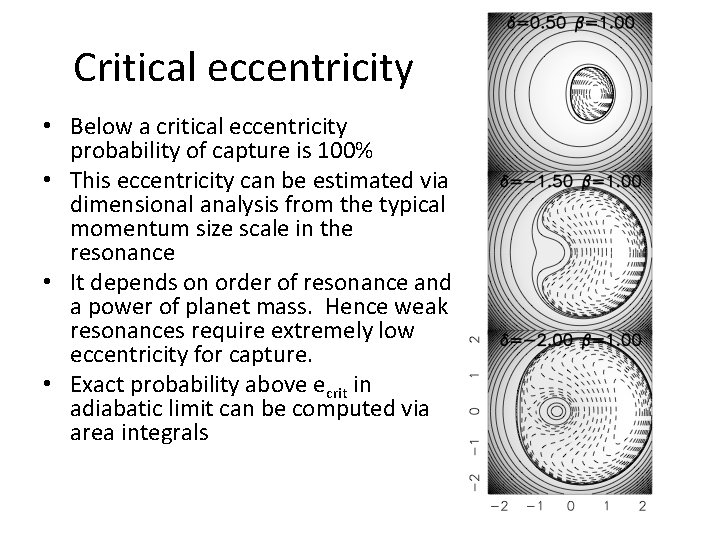Critical eccentricity • Below a critical eccentricity probability of capture is 100% • This eccentricity can be estimated via dimensional analysis from the typical momentum size scale in the resonance • It depends on order of resonance and a power of planet mass. Hence weak resonances require extremely low eccentricity for capture. • Exact probability above ecrit in adiabatic limit can be computed via area integrals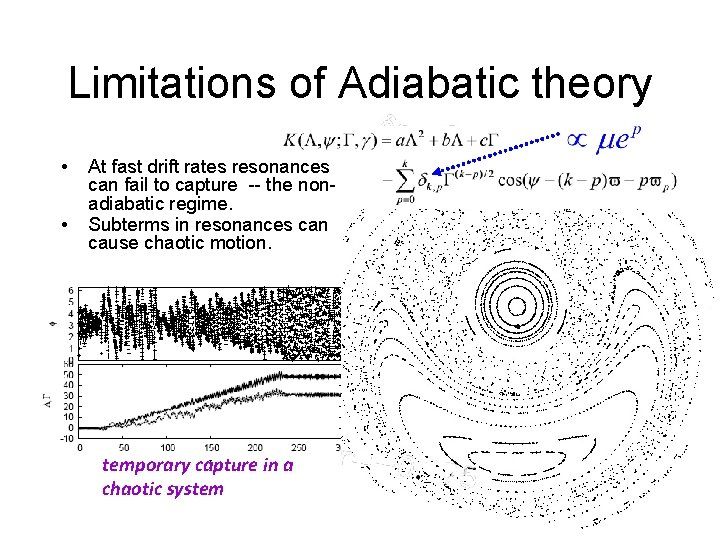Limitations of Adiabatic theory • • At fast drift rates resonances can fail to capture -- the nonadiabatic regime. Subterms in resonances can cause chaotic motion. temporary capture in a chaotic system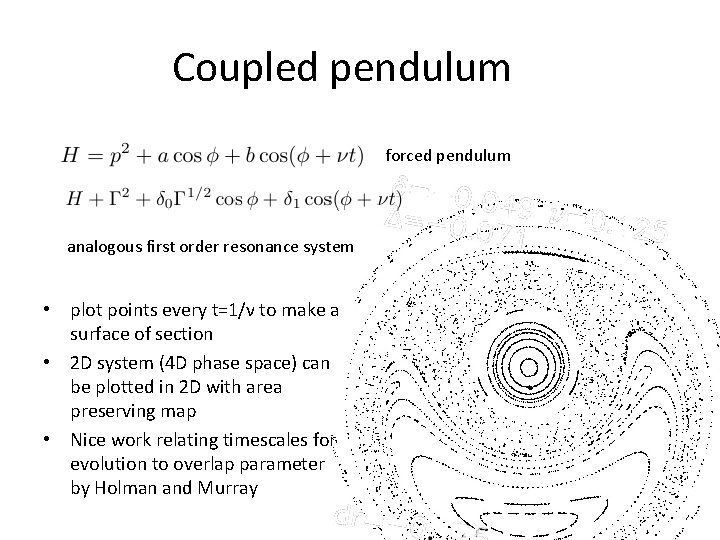Coupled pendulum forced pendulum analogous first order resonance system • plot points every t=1/ν to make a surface of section • 2 D system (4 D phase space) can be plotted in 2 D with area preserving map • Nice work relating timescales for evolution to overlap parameter by Holman and Murray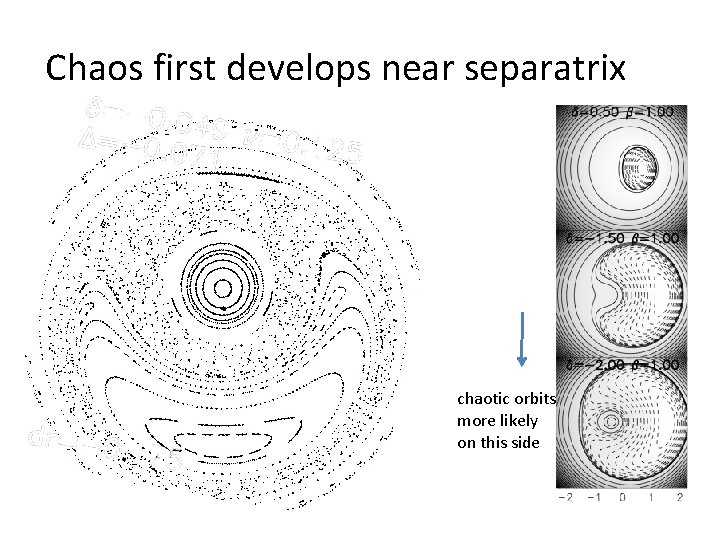Chaos first develops near separatrix chaotic orbits more likely on this side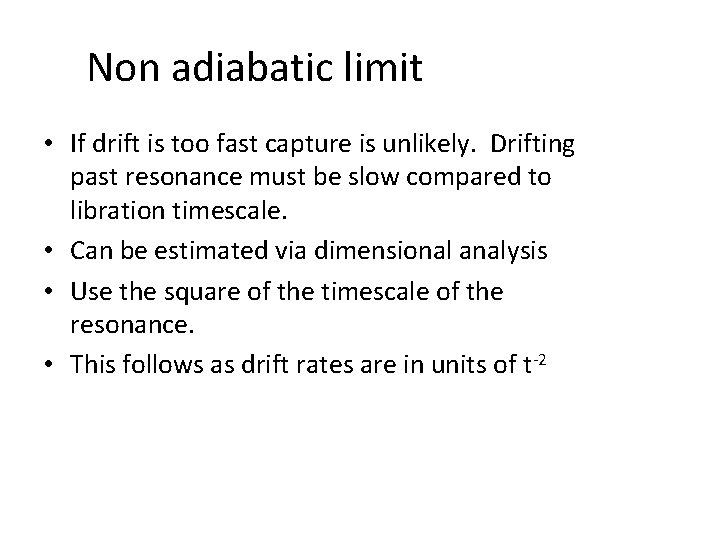Non adiabatic limit • If drift is too fast capture is unlikely. Drifting past resonance must be slow compared to libration timescale. • Can be estimated via dimensional analysis • Use the square of the timescale of the resonance. • This follows as drift rates are in units of t-2Reading • Morbidelli’s book Chap 1 • Murray and Dermott (solar system dynamics) Chap 8 • Yoshida, H. 1993, Celestial Mechanics and Dynamical Astronomy, 56, 27, “Recent Progress in the Theory and Application of Symplectic Integrators” • Duncan, Levison & Lee 1998, AJ, 116, 2067, “A multiple timestep symplectic algorithm for integrating close encounters” • Holman, Matthew J. , Murray, N. W. 1996, AJ, 112, 1278, “Chaos in High-Order Mean Resonances in the Outer Asteroid Belt” • Quillen, A. C. 2006, MNRAS, 365, 1367, `Reducing the probability of capture into resonance’ • Mustill & Wyatt 2011, MNRAS, 413, 554, `A general model of resonance capture in planetary systems: first- and second-order resonances’